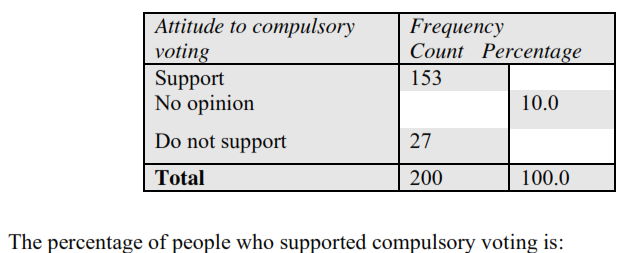# Chapter 1 - Displaying Data

Approved & Edited by ProProfs Editorial Team
The editorial team at ProProfs Quizzes consists of a select group of subject experts, trivia writers, and quiz masters who have authored over 10,000 quizzes taken by more than 100 million users. This team includes our in-house seasoned quiz moderators and subject matter experts. Our editorial experts, spread across the world, are rigorously trained using our comprehensive guidelines to ensure that you receive the highest quality quizzes.
| By Anthony Nunan
A
Anthony Nunan
Community Contributor
Quizzes Created: 159 | Total Attempts: 46,613
Questions: 30 | Attempts: 70Settings• 1.

### Two hundred people were asked about their attitude to compulsory voting (support, no opinion, do not support) and their age (in years).      The variables Attitude to compulsory voting and Age are:

• A.

Both categorical variables

• B.

Both numerical variables

• C.

A categorical and a numerical variable respectively

• D.

A numerical and a categorical variable respectively

• E.

Neither numerical nor categorical variables

• F.

I don't know

C. A categorical and a numerical variable respectively
Explanation
The variable "Attitude to compulsory voting" is categorical because it represents different categories or options (support, no opinion, do not support). The variable "Age" is numerical because it represents a quantitative measurement of people's ages in years.

Rate this question:

• 2.

### Two hundred people were asked about their attitude to compulsory voting (support, no opinion, do not support) and their age (in years).   The most appropriate way to graphically display the information about Age is to use a:

• A.

Dot plot

• B.

Bar chart

• C.

Histogram

• D.

Segmented bar chart

• E.

Back-to-back stem plot

• F.

I don't know

C. Histogram
Explanation
A histogram is the most appropriate way to graphically display the information about age. A histogram is a visual representation of the distribution of a dataset, where the data is divided into intervals or bins and the height of each bar represents the frequency or count of data points within that interval. In this case, the age data can be divided into different intervals (e.g., 0-10, 11-20, 21-30, etc.) and the height of each bar in the histogram will represent the number of people in each age group. This will provide a clear visual representation of the distribution of ages among the surveyed individuals.

Rate this question:

• 3.

### The variable number of people at a rock concert is a:

• A.

A continuous numerical variable

• B.

A discrete numerical variable

• C.

A continuous categorical variable

• D.

A discrete categorical variable

• E.

None of the above

• F.

I don't know

B. A discrete numerical variable
Explanation
A variable that represents the number of people at a rock concert would be considered a discrete numerical variable. This is because the number of people can only take on specific whole number values and cannot be divided into smaller increments.

Rate this question:

• 4.
• A.

10.0%

• B.

15.3%

• C.

27.0%

• D.

76.5%

• E.

90.0%

• F.

I don't know

D. 76.5%
• 5.
• A.

10

• B.

15

• C.

20

• D.

27

• E.

200

• F.

I don't know

C. 20
• 6.

### The age distribution for the population of Sassafras in 1986 is shown above   The percentage of people in Sassafras who were aged less than 20 years in 1986 was closest to:

• A.

15%

• B.

19%

• C.

20%

• D.

24%

• E.

34%

• F.

I don't know

E. 34%
Explanation
The correct answer is 34%. This can be determined by looking at the age distribution graph for the population of Sassafras in 1986. The graph shows that the largest bar represents the age group of 20-24 years, which means that there are more people in this age group compared to any other age group. Since the question asks for the percentage of people aged less than 20 years, we can infer that the percentage of people aged 20-24 years is higher than the percentage of people aged less than 20 years. Therefore, the percentage of people aged less than 20 years must be less than 34%, making 34% the closest option.

Rate this question:

• 7.

### The age distribution for the population of Sassafras in 1986 is shown above   In 1986, 1459 people lived in Sassafras. The number of residents under ten years of age was closest to:

• A.

19

• B.

146

• C.

186

• D.

277

• E.

729

• F.

I don't know

D. 277
• 8.

### The age distribution for the population of Sassafras in 1986 is shown above   The centre of the distribution lies between:

• A.

10 and 20

• B.

20 and 30

• C.

30 and 40

• D.

40 and 50

• E.

50 and 60

• F.

I don't know